NEET  >  Test: Newton’s First Law of Motion

# Test: Newton’s First Law of Motion

Test Description

## 10 Questions MCQ Test Physics Class 11 | Test: Newton’s First Law of Motion

Test: Newton’s First Law of Motion for NEET 2023 is part of Physics Class 11 preparation. The Test: Newton’s First Law of Motion questions and answers have been prepared according to the NEET exam syllabus.The Test: Newton’s First Law of Motion MCQs are made for NEET 2023 Exam. Find important definitions, questions, notes, meanings, examples, exercises, MCQs and online tests for Test: Newton’s First Law of Motion below.
Solutions of Test: Newton’s First Law of Motion questions in English are available as part of our Physics Class 11 for NEET & Test: Newton’s First Law of Motion solutions in Hindi for Physics Class 11 course. Download more important topics, notes, lectures and mock test series for NEET Exam by signing up for free. Attempt Test: Newton’s First Law of Motion | 10 questions in 10 minutes | Mock test for NEET preparation | Free important questions MCQ to study Physics Class 11 for NEET Exam | Download free PDF with solutions
 1 Crore+ students have signed up on EduRev. Have you?
Test: Newton’s First Law of Motion - Question 1

### If the net external force on the body is zero, its:

Detailed Solution for Test: Newton’s First Law of Motion - Question 1

Given, Fext = 0.

We know that F = ma

(where F is force, m is mass and a is acceleration)

As Mass (m) cannot be zero, therefore a = 0.

Test: Newton’s First Law of Motion - Question 2

### When a bull pulls a cart, the force that helps the bull to move forward is the force exerted by:

Detailed Solution for Test: Newton’s First Law of Motion - Question 2
• We know, every action has an equal and opposite reaction from Newton’s third law of motion.
• In this case, when the bull pulls a cart by a force say F, the cart also pulls the bull with an equal force F in the opposite direction and the net force is zero.
• But the bull is also pushing the ground at an angle by a force say F1.
• Now the ground also pushes the bull with a force of the same magnitude F1 but in opposite direction.
• The vertical component of the force balances with the weight of the bull and the cart but the unbalanced horizontal component of the force (if greater than frictional force) makes the bull move forward.
Test: Newton’s First Law of Motion - Question 3

### Dust can be removed out of a blanket using:

Detailed Solution for Test: Newton’s First Law of Motion - Question 3
• The Law of inertia also called Newton’s first law, postulates in physics that if a body is at rest or moving at a constant speed in a straight line, it will remain at rest or keep moving in a straight line at constant speed unless it is acted upon by force.
• According to the law of inertia, a particle tends to remain at rest or in uniform motion unless acted by an external force.
• When you jerk the blanket, it moves, but the dust particles remain at their original position.
• And finally, the dust gets separated. When we beat the blanket with a stick, it comes into motion.
• But the dust particles continue to be at rest due to inertia and get detached from the blanket.
Test: Newton’s First Law of Motion - Question 4

Which law governs the phenomenon that a tablecloth is pulled out from under a setting of chinaware without damaging it?

Detailed Solution for Test: Newton’s First Law of Motion - Question 4

According to Newton’s First  Law, the cloth is set in motion but the chinaware continues to remain in its state of rest; hence it is not damaged.

Additional Information: Newton's first law of motion is often stated as: An object at rest stays at rest and an object in motion stays in motion with the same speed and in the same direction unless acted upon by an unbalanced force.

Hence, option A is correct.

Test: Newton’s First Law of Motion - Question 5

Two masses are in the ratio 1:5. What is ratio of their inertia?

Detailed Solution for Test: Newton’s First Law of Motion - Question 5

Force of inertia = ma

Let the masses be 1x and 5x

Force of inertia for 1st body= 1x * a

Force of inertia for 2nd = 5x * a

Ratio= x * a / 5x * a = 1:5

Additional Information: The inability of a body to change it’s state of rest or uniform motion is called inertia.

Test: Newton’s First Law of Motion - Question 6

We slip on a rainy day due to ______.

Detailed Solution for Test: Newton’s First Law of Motion - Question 6
• It is usually seen that during rainy days we tend to slip because rainwater acts as a lubricant between our feet and the ground.

• This lubrication (rainwater) converts dry friction into fluid friction.

• That is, the friction between feet and ground reduces, making us slip.

Therefore, due to less friction, we slip on a rainy day.

Test: Newton’s First Law of Motion - Question 7

A 6 kg object is subject to three forces
F1 = 20+ 30j N
F2 = 8i - 50j N
F3 = 2i + 2j N
Find the acceleration of object.

Detailed Solution for Test: Newton’s First Law of Motion - Question 7

F = F1 + F2 + F3 = ( 20i + 30j) N+(8i - 50j) N+(2i +2 j) N

=(20+8+2)i+(30-50+2)j

F=30i - 18j

F = ma

Substituting F and m = 6 kg (given) in the above equation we get,

30i - 18j = 6*a

A = 5i - 3j

Hence (b) is correct choice

Test: Newton’s First Law of Motion - Question 8

Passengers in a bus lean forward as bus suddenly stops. This is due to

Detailed Solution for Test: Newton’s First Law of Motion - Question 8

When the bus moves, the passenger's body comes into a state of motion but when the bus stops the lower part of the body which is in contact with the floor comes into a state of rest whereas the upper part of the body still remains in the state of motion and because of this the upper part of the body falls in the forward direction. This is due to the presence of inertia.

Hence the correct answer is C.

Test: Newton’s First Law of Motion - Question 9

A particle of mass 'm' original at rest, is subjected to a force whose direction is constant but whose magnitude varies with according to the relation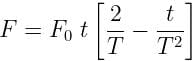,Where F0​ and T are constant.
Then speed of the particle after a time 2T is:

Detailed Solution for Test: Newton’s First Law of Motion - Question 9

Given:Speed of the particle after a time 2T,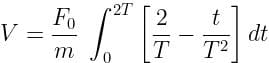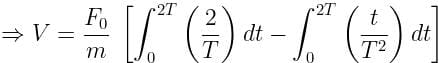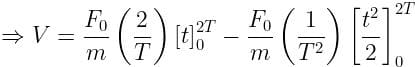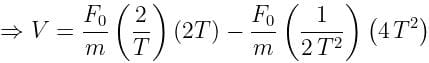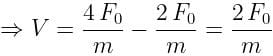Test: Newton’s First Law of Motion - Question 10

How is inertia used when riding a bicycle?

Detailed Solution for Test: Newton’s First Law of Motion - Question 10
• Newton’s first law of inertia states that an object at rest or in motion tends to stay at rest or moving with constant motion unless enacted upon by an unbalanced force.

• The law of inertia relates to the sport of cycling due to the fact that the cyclist is in constant motion when on the cycle.

• The motion of the bike is caused by the rider pressing down upon the pedals, which, in turn, enables the rider to accelerate on the bike.

• As well, the cyclist will continue to move unless enacted upon by an opposing unbalanced force, such as the force of friction on the bike tires, when the bike decelerates to a stop.

• The Law of Inertia is also displayed when the cyclist begins to move on the bicycle from a stop.

• The action is related to the concept of Inertia for the reason that the cyclist is applying an unbalanced force to the bicycle pedals when not in motion. As a result, the bicycle would gain acceleration and therefore begin to move.

Therefore the correct answer is B.

## Physics Class 11

127 videos|464 docs|210 tests
 Use Code STAYHOME200 and get INR 200 additional OFF Use Coupon Code
Information about Test: Newton’s First Law of Motion Page
In this test you can find the Exam questions for Test: Newton’s First Law of Motion solved & explained in the simplest way possible. Besides giving Questions and answers for Test: Newton’s First Law of Motion, EduRev gives you an ample number of Online tests for practice

## Physics Class 11

127 videos|464 docs|210 tests

### How to Prepare for NEET

Read our guide to prepare for NEET which is created by Toppers & the best Teachers